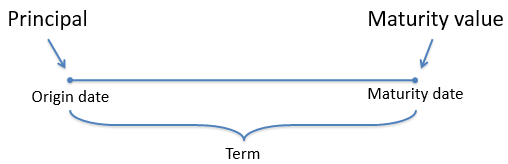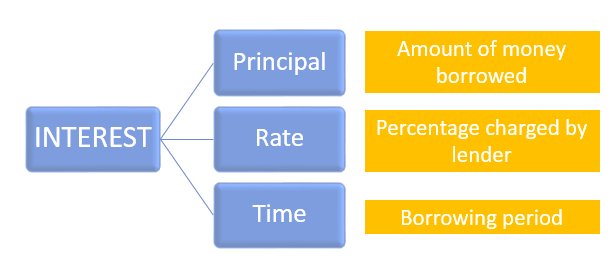Share It On :

# Simple interestDefinitions

Interest – An amount charged for the use of moneyPrincipal – The amount of money borrowed

Term – The length of the transaction period; it starts on the origin date and ends on the maturity date

Maturity value – the amount of money received at the end of the term; the sum of the principal and the interest earnedSimple interest – A type of interest wherein only the original principal earns interest for the duration of the term

Formula for simple interestInterest = Principal × Rate  ×  Time

Examples

Example 1

Find the interest earned after 3 years if  ₹ 12,000 is deposited in a savings account which earns 5% simple interest.

Solution :

I = PRT

I = 12000x .05x 3

I = 1800

Example 2

If you invested ₹ 200.00 in an account that paid simple interest of 4%, find the maturity value after 1.5 — years.

Solution

S = Principal + Interest

S= P+I

S = P + PRT

S = 200 + 200x.04x1.5

S=200+ 12

S = ₹ 212

Homework

1.  When invested at an annual interest rate of 6% an account earned 180.00 of simple interest in one year. How much money was originally invested in the account ?

2. A savings account is set up so that the simple interest on the investment is moved into a separate account at the end Of each year. If an Investment of 7,000 accumulate 910 Of interest in the account after 2 years, what was the annual simple interest rate on the saving account ?

3. Mr. Anand invested an amount of ₹ 13,900 divided in two different schemes A and B at the simple interest rate of 14% p.a. and 11% p.a. respectively. If the total amount of simple interest earned in 2 years be Rs. 3508, what was the amount invested in Scheme B?

4.  Asmita took a loan of ₹ 1200 with simple interest for as many years as the rate of interest. If she paid ₹  432 as interest at the end of the loan period, what was the rate of interest?

5. There was simple interest of ₹  4016.25 on a principal amount at the rate of 9%p.a. in 5 years. Find the principal amount